cancel
Showing results for
Did you mean: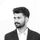# How do we conditionally format bubbles in the Power BI scatter chart based on the trend line value?

First we have to create a DAX measure to get the trend line values. Since February 2023 Power BI update, we have two new statistical functions LINEST() and LINESTX(). Using these functions we can easily create DAX measure to get the trend line.

Sample dataBelow is the trend line measure

``````TrendLine =
VAR _linest_output =
LINESTX (
ALLSELECTED ( 'Table' ),
'Table'[Variable 2],
'Table'[Variable 1],
TRUE
)
VAR _slope =
MAXX ( _linest_output, [Slope1] )
VAR _intercept =
MAXX ( _linest_output, [Intercept] )
VAR _result =
SUMX (
DISTINCT ( 'Table'[Variable 1] ),
_intercept + _slope * 'Table'[Variable 1]
)
RETURN
_result``````

You may notice an error indication in the variables _slope and _intercept.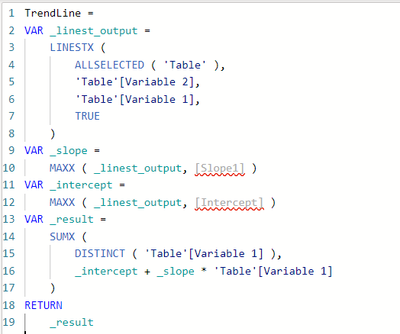But you can ignore it. Hope it will be resolved in the upcoming Power BI update. Anyway, the measure will work as expected.

Next, we need to create a measure for conditional formatting.

Below is the sample measure.

``````Color =
IF ( SUM ( 'Table'[Variable 2] ) > [TrendLine], "Red", "Green" )``````

You can customize the logic based on your need. Here we are just coloring the bubble as red if the Y axis value is higher than trend line, else color will be green.

Now you can use scatter chart from the visualization pane. In this example, you can use [Variable 1] column into X axis, [Variable 2] into Y axis and [Category] column into Values.

If you use Legend property, conditional formatting will not work.

Next you can navigate to visual formating pane. Under Markers option, you can click on fx icon near to color section.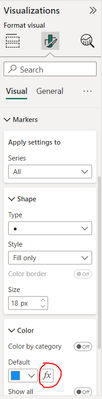Then you can select Format style as Field value, select newly created measure (Color) in What field should we base this on? and click ok.Then you will get the result as below.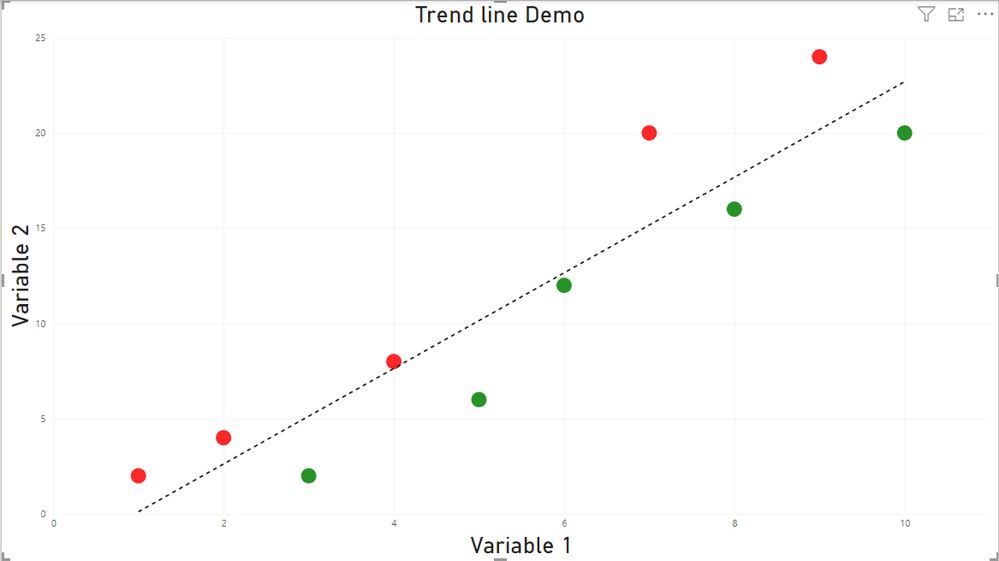To enable the trend line in the scatter chart, you can navigate into analytics pane and turn on trend line.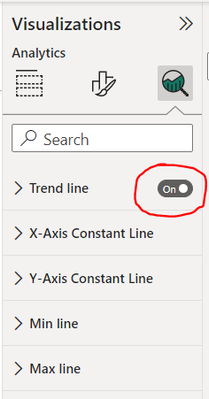Regards,

Nandu KrishnaThanks Nandu really good one.

This is really helpful.. Thanks Nandu!!

I have another question ? Is there way that we can control the opacity of scatter plot so that overlapping data points are more visible?@Manmeet Opacity can be controlled by using HEX value.

Calculated Table

``Intensity = GENERATESERIES(0, 100, 5)``

Measure

``Intensity Value = SELECTEDVALUE('Intensity'[Value], 100)``

Measure

``````Color =
VAR _defaultColor = "#1565C0"
VAR _transparencyMapping =
DATATABLE (
"Perc", INTEGER,
"Sufix", STRING,
{
{ 100, "FF" },
{ 95, "F2" },
{ 90, "E6" },
{ 85, "D9" },
{ 80, "CC" },
{ 75, "BF" },
{ 70, "B3" },
{ 65, "A6" },
{ 60, "99" },
{ 55, "8C" },
{ 50, "80" },
{ 45, "73" },
{ 40, "66" },
{ 35, "59" },
{ 30, "4D" },
{ 25, "40" },
{ 20, "33" },
{ 15, "26" },
{ 10, "1A" },
{ 5, "0D" },
{ 0, "00" }
}
)
VAR _color =
CONCATENATEX (
FILTER ( _transparencyMapping, [Perc] = [Intensity Value] ),
_defaultColor & [Sufix]
)
RETURN
_color``````Nice job!

Top Kudoed Posts
Latest Articles
Archives
Polls
What is your favorite Power BI feature release for September 2023?